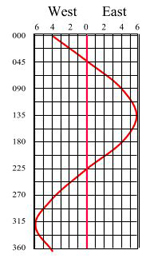# Compass north – YM

## Preparation

Before reading this page you will need to have a copy of the training almanac and chart (and a real ones if possible).

You will need to be happy converting between magnetic and true north.

## Why is this important?

The magnetic effect of our boat can deflect our compass. It is possible to allow for this error in the compass bearing.

### Deviation & compass bearings

All the concepts that were covered in the Day Skipper course are still the same on the Coastal Skipper course with one additional problem thrown in.

A magnetic compass will generally point in the direction of magnetic north. However, if other magnetic, metal or electrical objects are brought close to the compass they will deflect the compass.

We can avoid this in many cases be removing the problem and being careful when positioning equipment. For example, not positioning the VHF (with a large magnetic in the speaker) near the radio, this is a common problem in RIBS when all instrutments are contained in a small area.

Other magnetic objects cannot be removed, for examples the engine, cooker or event the hull on a steel boat. These have a constant effect on the compass which is known as deviation.

The one complication with deviation is that it varies depending on which way you point the boat.

###Allowing for deviation

To find out the effect of deviation we must “swing the compass” of our boat. For details of how this is done see this Sail Train article [swinging a compass…]

Once your compass has been swung you will have a compass deviation card (like the one on the right), these are different from boat to boat but will generally follow a sine wave.

For example, on the compass card to the right you can see that if your boat in on a course of 090o(vertical scale) the deviation which needs to be applied is 4oE.

You can download a blank Deviation Card from the Cockpit Cards website [Cockpit Cards]

### Method 1 – Calculation

First recall the following saying:

“Variation West, Compass Best – Variation East, Compass Least”

Translated this means, when the variation (or deviation) is west the magnetic (or compass) bearing is biggest (best); when the variation (or deviation) is east the magnetic (or compass) bearing is smallest (least).

You now just need to know that the order of adjustments is as follows (move from left to right or right to left):

 True Bearing Variation (from the chart) Magnetic Bearing Deviation (from the compass card) Compass Bearing

This means:

True to Compass: From the true bearing you must first make the variation adjustment (as before) to find the magnetic bearing and apply the deviation adjustment (finding the deviation on the compass card) to find the compass bearing.

So for example: If you read a true bearing is 340o from the chart and the variation is 4oW then, in this case, the magnetic bearing is the largest (best) so we add 4o so we get a magnetic bearing of 344o. From the compass card the deviation is 5oW to the nearest whole number. In this case the compass bearing is best so we add 5o to get a compass bearing of 349o

 True Bearing Variation (from the chart) Magnetic Bearing Deviation (from the compass card) Compass Bearing 340o 4oW 344o 5oW 349o

Compass to True: From the compass bearing you must first make the deviation adjustment to find the magnetic bearing and apply the variation adjustment to find the true bearing.

So for example: If the compass bearing is 056o we can read off the compass card a deviation of 1oE so the compass bearing will be “least” so we add 1o to make a magnetic bearing of 057o the variation is 7oW then in this case the magnetic bearing is the “best” so we subtract 7o so we get a true bearing of 050o.

 True Bearing Variation (from the chart) Magnetic Bearing Deviation (from the compass card) Compass Bearing 050o 7oW 057o 1oE 056o

First recall the following saying:

Translated this means, if you have the compass bearing you should add east deviation to get the true bearing.

Therefore you can reverse the above statement so that for an east deviation you subtract the variation to translate from true to compass.

You now just need to know that the order of adjustments is as follows (move from left to right or right to left):

 True Bearing Variation (from the chart) Magnetic Bearing Deviation (from the compass card) Compass Bearing

This means:

True to Compass: From the true bearing you must first make the variation adjustment (as before) to find the magnetic bearing and apply the deviation adjustment (finding the deviation on the compass card) to find the compass bearing.

So for example: If you read a true bearing is 340o from the chart and the variation is 4oW then in this case we add 4o so we get a magnetic bearing of 344o. From the compass card the deviation is 5oW to the nearest whole number. Therefore, we also add 5o to get a compass bearing of 349o

 True Bearing Variation (from the chart) Magnetic Bearing Deviation (from the compass card) Compass Bearing 340o 4oW 344o 5oW 349o

Compass to True: From the compass bearing you must first make the deviation adjustment to find the magnetic bearing and apply the variation adjustment to find the true bearing.

So for example: If the compass bearing is 056o we can read off the compass card a deviation of 1oE so we add 1o to make a magnetic bearing of 057o. The variation is 7oW then therefore we subtract 7o so we get a true bearing of 050o.

 True Bearing Variation (from the chart) Magnetic Bearing Deviation (from the compass card) Compass Bearing 050o 7oW 057o 1oE 056o